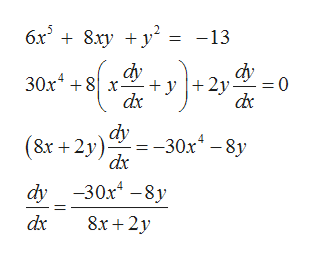# Find the slope of the tangent line to the curve defined by6x5 + 8xy +y2 = -13 at (-1,1).The slope of the curve at the point (-1,1) is ?

Question

Find the slope of the tangent line to the curve defined by

6x+ 8xy +y= -13 at (-1,1).

The slope of the curve at the point (-1,1) is ?

check_circleExpert Solution
Step 1

The slope of tangent line to the curve f(x,y)=0 at (a,b) is the value of dy/dx at (a,b)

Step 2

We differentiate both sides of given equation of curve to find dy/dxhelp_outlineImage Transcriptionclose6x8.xy y -13 dy dy 30x48 - 2y dc 0 = dx dy 30x4-8y (8х + 2у) dx dy 30x-8y 8x+2y dx fullscreen
Step 3

Then we substitute x=-1 and y=1 in dy/dx to get the value o...

### Want to see the full answer?

See Solution

#### Want to see this answer and more?

Solutions are written by subject experts who are available 24/7. Questions are typically answered within 1 hour*

See Solution
*Response times may vary by subject and question
Tagged in

### Calculus